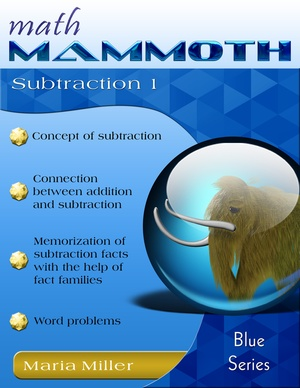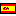^
You are here: HomeBlue Series → Subtraction 1

# Math Mammoth Subtraction 189 pages

## Prices & ordering

Printed copy \$10.50

(credit cards, Google Pay, Paypal, PO)

Printed copy £5.64
US shipping availableEste libro en español

Math Mammoth Subtraction 1 is a worktext that covers the concept of subtraction, the relationship between addition and subtraction, and the various meanings of subtraction.

In the first lesson, Subtraction Is Taking Away, the child learns the basic meaning of subtraction as taking away objects, and learns to write subtractions from an illustration where some objects are crossed out.

In the next lesson, the child counts down to subtract, which ties in subtraction with the number line. This is a transitional strategy to solve subtraction problems, because later students will learn more efficient ways to subtract, but it is important conceptually.

The following lesson, Subtraction and Addition in the Same Picture, begins the study of the relationship between addition and subtraction. This concept will span several lessons. This first lesson presents two sets of objects, such as blue and white balls, and the student writes both an addition sentence and a subtraction sentence from this illustration.

The lesson When Can You Subtract? concentrates on the idea that some subtractions, such as 4 − 5, are meaningless when you think of taking away. The child also makes subtraction patterns in this lesson.

Then we continue studying the connection between addition and subtraction in the lesson Two Subtractions from One Addition. As an example, the child writes both 8 − 3 = 5 and 8 − 5 = 3 from the addition 3 + 5 = 8. This idea ties in with fact families, a concept that is coming up soon.

In the lesson Two Parts — One Total, we study word problems that do not involve the idea of taking away but have two parts making up a total. For example, if there are 10 flowers of which some are white and some are red, and seven of them are white, how many are red?

Then we study fact families, which means writing two additions and two subtractions using the same three numbers.

In the lesson How Many More? students find how many more or how many fewer objects one person has than the other by drawing the objects. This lesson can easily be done with manipulatives if desired.

In the very next lesson, “How Many More” Problems and Differences, we continue the theme, this time writing a missing addend addition for problems that ask “how many more.” For example, Veronica has 4 marbles and Ann has 6. We write the missing addend sentence 4 + ___ = 6 to find how how many more Ann has. In the next lesson the child then learns to write subtraction sentences for such problems.

The PDF version of this book can be filled in on a computer, phone, or tablet, using the annotation tools found in many PDF apps. See more.

While this book is not tied to any grade level, it is most suitable for first grade.

Check out also these free subtraction games and activities at our site: You might also be interested in the following books and worksheet resources:
• Math Mammoth Addition 1 – a worktext about addition concept within 0-10, sum families (sums with 7, 8, etc.), and word problems (grade 1).
• Math Mammoth Add & Subtract 2-A – a worktext about "easy" additions and subtractions within 0-100, memorizing the basic addition & subtraction facts within 0-18, and word problems (grades 1-2).
• Math Mammoth Place Value 1 – a worktext about two-digit numbers (place value), comparing, rounding, even & odd, and graphs (grade 1).
• Free subtraction worksheets for grade 1 – includes single-digit subtraction, double-digit subtraction, subtracting multiples of ten, and missing number subtraction problem worksheets.

## Customer Testimonials

Maria,

My 4 year old just finished Subtraction 1. We've done Addition 1 and Place Value 1 this fall as well. He loves it! I found myself frustrated with Saxon Math K and found Math Mammoth last summer. We're going to breeze through Light Blue 1 this spring and have fun committing his math facts to memory. Thank you for a simple straightforward math program!

Amanda Pelser
http://thepelsers.com

## Better Yet - Bundle Deals!

*BONUS*: Buy any bundle listed above, and get the fully upgraded Soft-Pak software for FREE (includes 4 math and 2 language arts programs, complete with on screen and printable options).

## How and where to order

You can buy Math Mammoth books at:

• Here at MathMammoth.com website — simply use the "Add to cart" buttons you see on the product pages.
• Rainbow Resource carries printed copies for the Light Blue series books, plus several CDs (Light Blue and Blue series).
• Homeschool Buyers Club offers download versions of the Light Blue series, plus the Blue series bundle.
• Homeschool Planet sells the digital versions of the main curriculum and lesson plans to go with them.
• Lulu sells printed copies for most of the Math Mammoth materials (various series).

By purchasing any of the books, permission IS granted for the teacher (or parent) to reproduce this material to be used with his/her students in a teaching situation; not for commercial resale. However, you are not permitted to share the material with another teacher.

In other words, you are permitted to make copies for the students/children you are teaching, but not for other teachers' usage.

Math Mammoth books are PDF files. I recommend you use Adobe Reader to view them, including if you use a Mac. You can try other PDF viewers, but they may at times either omit or mess up some of the images.
WAIT!

Receive my monthly collection of math tips & resources directly in your inbox — and get a FREE Math Mammoth book!You can unsubscribe at any time.

### Math Mammoth Tour

Confused about the different options? Take a virtual email tour around Math Mammoth! You'll receive:

An initial email to download your GIFT of over 400 free worksheets and sample pages from my books. Six other "TOURSTOP" emails that explain the important things and commonly asked questions concerning Math Mammoth curriculum. (Find out the differences between all these different-colored series!)

This way, you'll have time to digest the information over one or two weeks, plus an opportunity to ask me personally about the curriculum.
A monthly collection of math teaching tips & Math Mammoth updates (unsubscribe any time)### "Mini" Math Teaching Course

This is a little "virtual" 2-week course, where you will receive emails on important topics on teaching math, including:

- How to help a student who is behind
- Troubles with word problems
- Teaching multiplication tables
- Why fractions are so difficult
- The value of mistakes
- Should you use timed tests
- And more!

A GIFT of over 400 free worksheets and sample pages from my books right in the very beginning.A monthly collection of math teaching tips & Math Mammoth updates (unsubscribe any time)
Enter your email to receive math teaching tips, resources, Math Mammoth news & sales, humor, and more! I tend to send out these tips about once monthly, near the beginning of the month, but occasionally you may hear from me twice per month (and sometimes less often).• A GIFT of over 400 free worksheets and sample pages from my books.